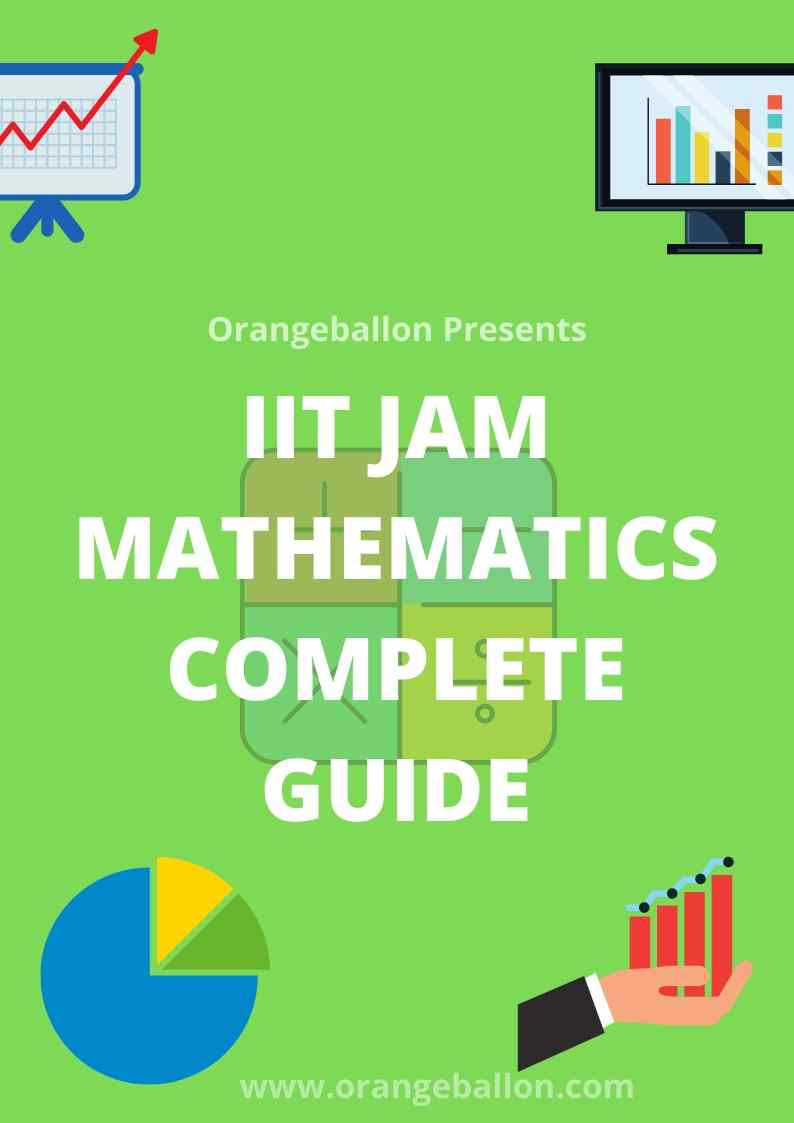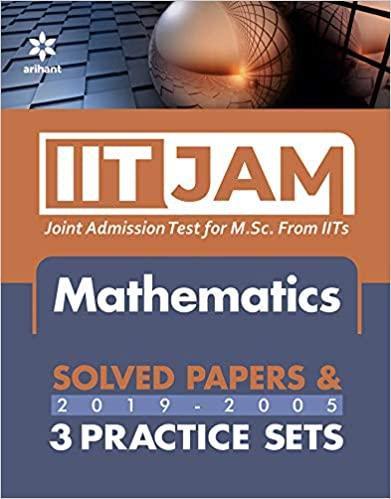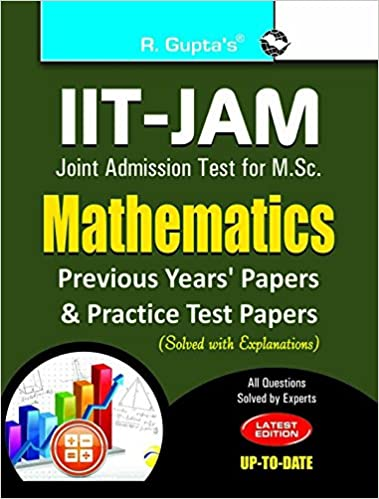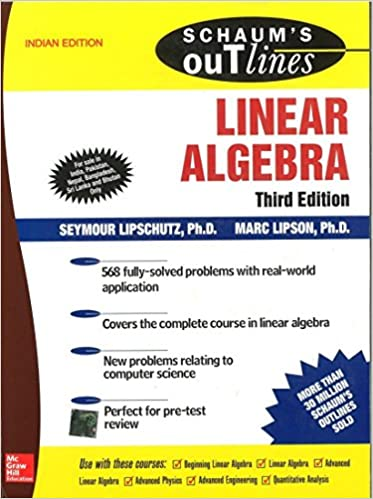`IIT JAM Mathematics is one of the best MSc exams in the country. Every year more than 14000 students appear for this exam for less than 500 seats. In this post, we will discuss complete guide of IIT JAM Mathematics paper including syllabus, the pattern of test paper, last 4 year papers of IIT JAM Mathematics, opening and closing rank, the number of seats in IITs for mathematics and at the end some useful tips and books to prepare for IIT JAM Mathematics.IIT JAM Examination is conducted in six subjects, also referred to as Test Papers; Biotechnology (BT), Chemistry (CY), Geology (GG), Mathematics (MA), Mathematical Statistics (MS) and Physics (PH).IIT JAM Mathematics comes with a bright future scope. Data scientist, data analyst, Researcher, teaching, software engineer are some of the jobs offered to Mathematics students from IITs. Content:1. IIT JAM Syllabus for mathematics2. The pattern of IIT JAM mathematics Test Papers3. IIT JAM Mathematics previous year papers4. Opening and Closing Ranks for IIT JAM 20205. IIT JAM Mathematics  Seats from IITs6. Useful Tips for preparation of IIT JAM exam MathematicsIIT JAM Syllabus for Mathematics:Sequences and Series of Real Numbers: Sequence of real numbers, the convergence of sequences, bounded and monotone sequences, convergence criteria for sequences of real numbers, Cauchy sequences, subsequences, Bolzano-Weierstrass theorem. Series of real numbers, absolute 28 convergence, tests of convergence for series of positive terms – comparison test, ratio test, root test; Leibniz test for convergence of alternating series. Functions of One Real Variable: Limit, continuity, intermediate value property, differentiation, Rolle’s Theorem, mean value theorem, L'Hospital rule, Taylor's theorem, maxima, and minima. Functions of Two or Three Real Variables: Limit, continuity, partial derivatives, differentiability, maxima, and minima. Integral Calculus: Integration as the inverse process of differentiation, definite integrals, and their properties, fundamental theorem of calculus. Double and triple integrals, change of order of integration, calculating surface areas and volumes using double integrals, calculating volumes using triple integrals. Differential Equations: Ordinary differential equations of the first order of the form y'=f(x,y), Bernoulli’s equation, exact differential equations, integrating factor, orthogonal trajectories, homogeneous differential equations, variable separable equations, linear differential equations of second order with constant coefficients, Method of variation of parameters, Cauchy-Euler equation. Vector Calculus: Scalar and vector fields, gradient, divergence, curl, line integrals, surface integrals, Green, Stokes, and Gauss theorems. Group Theory: Groups, subgroups, Abelian groups, non-Abelian groups, cyclic groups, permutation groups, normal subgroups, Lagrange's Theorem for finite groups, group homomorphisms, and basic concepts of quotient groups.Linear Algebra: Finite dimensional vector spaces, linear independence of vectors, basis, dimension, linear transformations, matrix representation, range space, null space, rank-nullity theorem. Rank and inverse of a matrix, determinant, solutions of systems of linear equations, consistency conditions, eigenvalues, and eigenvectors for matrices, Cayley-Hamilton theorem.Real Analysis: Interior points, limit points, open sets, closed sets, bounded sets, connected sets, compact sets, completeness of R. Power series (of a real variable), Taylor’s series, radius and interval of convergence, term-wise differentiation and integration of power series.IIT JAM Mathematics Topic-wise marks DistributionTopicsAvg. Marks DistributionDifferential Calculus23 MarksLinear Algebra13 MarksSequence and Series12 MarksDifferential Equation12 MarksIntegral Calculus11 MarksGroup Theory11 MarksVector Calculus10 MarksReal Analysis8 MarksThe pattern of IIT JAM Mathematics Test Papers:The JAM 2020 Examination for all the six test papers will be carried out as ONLINE Computer Based Test (CBT) where the candidates will be shown the questions in a random sequence on a computer screen. For all the six test papers, the duration of the examination will be 3 hours. The medium for all the test papers will be English only.There will be a total of 60 questions carrying 100 marks. The entire paper will be divided into three sections, A, B, and C. All sections are compulsory. Questions in each section will be of different types as given below:Section–A contains a total of 30 Multiple Choice Questions (MCQs) involving 10 questions of one mark each and 20 questions of two marks each.Each MCQ has four choices out of which only one choice is the correct answer. Candidates can mark the answer by clicking the choice.Section–B contains a total of 10 Multiple Select Questions (MSQs) carrying two marks each. Each MSQ is similar to MCQ but with the difference that MSQ may have one or more than one correct choice(s) out of the four given choices. The candidate gets full credit only if he/she selects all the correct answer(s) only and no wrong answers. Candidates can mark the answer(s) by clicking the choice(s).Section–C contains a total of 20 Numerical Answer Type (NAT) questions involving 10 questions of one mark each and 10 questions of two marks each. For these NAT type questions, the answer is a signed real number, which needs to be entered using the virtual numeric keypad on the monitor. No choices will be shown for NAT questions.In all sections, questions not attempted will result in zero marks. In Section-A (MCQ), the wrong answer will result in negative marks. For each wrong answer to 1 mark questions, 1/3 mark will be deducted and similarly, for each wrong answer to 2 marks questions, 2/3 mark will be deducted.In Section-B (MSQ), there are no negative and no partial marking provisions. There is no negative marking in Section-C (NAT) as well.There is a provision of using the online virtual calculator. The candidates, therefore, should not bring any calculator with them.Mobile phones or any other electronic devices are strictly prohibited inside the examination hall. Charts, graph sheets, and tables are also NOT allowed inside the examination hall.A scribble pad will be provided for rough work and this has to be returned back at the end of the examination.The candidates are required to select the answer for MCQ and MSQ type questions using the mouse. The answer for NAT questions can be entered using a virtual numeric keypad (the keyboard of the computer will be disabled).At the end of the 3 hours, the computer will automatically end the examination.IIT JAM Mathematical Mathematics previous year papers:1. IIT JAM Mathematics Question Paper year 20172. IIT JAM Mathematics Question Paper year 20183. IIT JAM Mathematics Question Paper year 20194. IIT JAM Mathematics Question Paper year 2020 (answer key)IIT JAM Mathematics Seats from IITsThe competition is very high for IIT JAM Mathematics as  there are limited seats in IITs for mathematics and there are 14000+ candidates who apply for the IIT JAM Mathematics every year for over 450+ seatsOpening and Closing Ranks for IIT JAM 2020:1. JAM 2019 Round 1 (First) Opening and Closing Ranks2. JAM 2019 Round 2 (Second) Opening and Closing Ranks3. JAM 2019 Round 3 (Final) Opening and Closing RanksUseful Posts for preparation of IIT JAM exam:10 Tips and tricks to prepare for entrance examsPomodoro Technique: How to study more in less time?6 ways to boost your performance in the examUseful Books for IIT JAM Mathematics papers:Some topic wise IIT JAM Mathematics Reference Books that will help you to prepare better. These are the books referred by toppers of IIT JAM mathematics.  Integral Calculus: F. Ayres (Schaum’s), Gorakh Prasad Vector Calculus: Murray R. Spiegel (Schaum’s), A.R.Vasishtha Linear Algebra: Seymour Lipschitz (Schaum’s), H. Anton, A.R.Vasishtha. Ordinary Differential Equation: Peter J. Collins, G.F. Simmons, M.D. Raisinghania. Principle of Real Analysis: S. C. Malik. Real Analysis: Book by H. L. Royden, Book by Shanti Narayan Modern Algebra: A. R. Vasishtha, University Algebra by N. S. Gopalakrishnan.More about IIT JAM 2021: IIT JAM 2021 Exam Dates, Syllabus, Application Form, ResultAll the best for your IIT JAM Exam 2021.`